# getEntry

Get entry contents from `SimBiology.Scenarios` object

## Syntax

``entryStruct = getEntry(sObj,entryNameOrIndex)``
``entryStruct = getEntry(sObj,entryIndex,subIndex)``

## Description

example

````entryStruct = getEntry(sObj,entryNameOrIndex)` returns a structure containing the contents of the entry (or subentry) specified by `entryNameOrIndex`.```

example

````entryStruct = getEntry(sObj,entryIndex,subIndex)` returns a structure containing the contents of a subentry specified by `entryIndex` and `subIndex`.```

## Examples

collapse all

Load the model of glucose-insulin response. For details about the model, see the Background section in Simulating the Glucose-Insulin Response.

`sbioloadproject('insulindemo','m1');`

The model contains different parameter values and initial conditions that represents different insulin impairments (such as Type 2 diabetes, low insulin sensitivity, and so on) stored in five variants.

`variants = getvariant(m1)`
```variants = SimBiology Variant Array Index: Name: Active: 1 Type 2 diabetic false 2 Low insulin se... false 3 High beta cell... false 4 Low beta cell ... false 5 High insulin s... false ```

Suppress an informational warning that is issued during simulations.

`warnSettings = warning('off','SimBiology:DimAnalysisNotDone_MatlabFcn_Dimensionless');`

Select a dose that represents a single meal of 78 grams of glucose.

`singleMeal = sbioselect(m1,'Name','Single Meal');`

Create a `Scenarios` object to represent different initial conditions combined with the dose. That is, create a `scenario` object where each variant is paired (or combined) with the dose, for a total of five simulation scenarios.

```sObj = SimBiology.Scenarios; add(sObj,'cartesian','variants',variants); add(sObj,'cartesian','dose',singleMeal)```
```ans = Scenarios (5 scenarios) Name Content Number ________ ___________________ ______ Entry 1 variants SimBiology variants 5 x Entry 2 dose SimBiology dose 1 See also Expression property. ```

`sObj` contains two entries. Use the `generate` function to combine the entries and generate five scenarios. The function returns a scenarios table, where each row represents a scenario and each column represents an entry of the `Scenarios` object.

`scenariosTbl = generate(sObj)`
```scenariosTbl=5×2 table variants dose ______________________ _________________________ 1x1 SimBiology.Variant 1x1 SimBiology.RepeatDose 1x1 SimBiology.Variant 1x1 SimBiology.RepeatDose 1x1 SimBiology.Variant 1x1 SimBiology.RepeatDose 1x1 SimBiology.Variant 1x1 SimBiology.RepeatDose 1x1 SimBiology.Variant 1x1 SimBiology.RepeatDose ```

Change the entry name of the first entry.

`rename(sObj,1,'Insulin Impairements')`
```ans = Scenarios (5 scenarios) Name Content Number ____________________ ___________________ ______ Entry 1 Insulin Impairements SimBiology variants 5 x Entry 2 dose SimBiology dose 1 See also Expression property. ```

Create a `SimFunction` object to simulate the generated scenarios. Use the `Scenarios` object as the input and specify the plasma glucose and insulin concentrations as reponses (outputs of the function to be plotted). Specify `[]` for the dose input argument since the `Scenarios` object already has the dosing information.

`f = createSimFunction(m1,sObj,{'[Plasma Glu Conc]','[Plasma Ins Conc]'},[])`
```f = SimFunction Parameters: Name Value Type Units _________________________ ______ _____________ ___________________________________________ {'Plasma Volume (Glu)' } 1.88 {'parameter'} {'deciliter' } {'k1' } 0.065 {'parameter'} {'1/minute' } {'k2' } 0.079 {'parameter'} {'1/minute' } {'Plasma Volume (Ins)' } 0.05 {'parameter'} {'liter' } {'m1' } 0.19 {'parameter'} {'1/minute' } {'m2' } 0.484 {'parameter'} {'1/minute' } {'m4' } 0.1936 {'parameter'} {'1/minute' } {'m5' } 0.0304 {'parameter'} {'minute/picomole' } {'m6' } 0.6469 {'parameter'} {'dimensionless' } {'Hepatic Extraction' } 0.6 {'parameter'} {'dimensionless' } {'kmax' } 0.0558 {'parameter'} {'1/minute' } {'kmin' } 0.008 {'parameter'} {'1/minute' } {'kabs' } 0.0568 {'parameter'} {'1/minute' } {'kgri' } 0 {'parameter'} {'1/minute' } {'f' } 0.9 {'parameter'} {'dimensionless' } {'a' } 0 {'parameter'} {'1/milligram' } {'b' } 0.82 {'parameter'} {'dimensionless' } {'c' } 0 {'parameter'} {'1/milligram' } {'d' } 0.01 {'parameter'} {'dimensionless' } {'kp1' } 2.7 {'parameter'} {'milligram/minute' } {'kp2' } 0.0021 {'parameter'} {'1/minute' } {'kp3' } 0.009 {'parameter'} {'(milligram/minute)/(picomole/liter)' } {'kp4' } 0.0618 {'parameter'} {'(milligram/minute)/picomole' } {'ki' } 0.0079 {'parameter'} {'1/minute' } {'[Ins Ind Glu Util]' } 1 {'parameter'} {'milligram/minute' } {'Vm0' } 2.5129 {'parameter'} {'milligram/minute' } {'Vmx' } 0.047 {'parameter'} {'(milligram/minute)/(picomole/liter)' } {'Km' } 225.59 {'parameter'} {'milligram' } {'p2U' } 0.0331 {'parameter'} {'1/minute' } {'K' } 2.28 {'parameter'} {'picomole/(milligram/deciliter)' } {'alpha' } 0.05 {'parameter'} {'1/minute' } {'beta' } 0.11 {'parameter'} {'(picomole/minute)/(milligram/deciliter)'} {'gamma' } 0.5 {'parameter'} {'1/minute' } {'ke1' } 0.0005 {'parameter'} {'1/minute' } {'ke2' } 339 {'parameter'} {'milligram' } {'Basal Plasma Glu Conc'} 91.76 {'parameter'} {'milligram/deciliter' } {'Basal Plasma Ins Conc'} 25.49 {'parameter'} {'picomole/liter' } Observables: Name Type Units _____________________ ___________ _______________________ {'[Plasma Glu Conc]'} {'species'} {'milligram/deciliter'} {'[Plasma Ins Conc]'} {'species'} {'picomole/liter' } Dosed: TargetName TargetDimension __________ _____________________ {'Dose'} {'Mass (e.g., gram)'} TimeUnits: hour ```

Simulate the model for 24 hours and plot the simulation data. The data contains five runs, where each run represents a scenario in the Scenarios object.

```sd = f(sObj,24); sbioplot(sd)```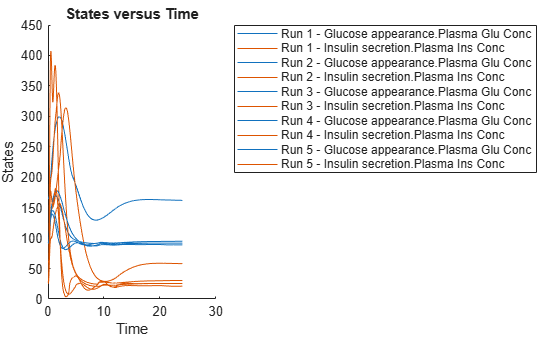```ans = Axes (SbioPlot) with properties: XLim: [0 30] YLim: [0 450] XScale: 'linear' YScale: 'linear' GridLineStyle: '-' Position: [0.0956 0.1100 0.2509 0.8150] Units: 'normalized' Show all properties ```

If you have Statistics and Machine Learning Toolbox™, you can also draw sample values for model quantities from various probability distributions. For instance, suppose that the parameters `Vmx` and `kp3`, which are known for the low and high insulin sensitivity, follow the lognormal distribution. You can generate sample values for these parameters from such a distribution, and perform a scan to explore model behavior.

Define the lognormal probability distribution object for `Vmx`.

`pd_Vmx = makedist('lognormal')`
```pd_Vmx = LognormalDistribution Lognormal distribution mu = 0 sigma = 1 ```

By definition, the parameter `mu` is the mean of logarithmic values. To vary the parameter value around the base (model) value of the parameter, set `mu` to `log(``model_value``)`. Set the standard deviation (sigma) to 0.2. For a small sigma value, the mean of a lognormal distribtion is approximately equal to `log(``model_value``)`. For details, see Lognormal Distribution (Statistics and Machine Learning Toolbox).

```Vmx = sbioselect(m1,'Name','Vmx'); pd_Vmx.mu = log(Vmx.Value); pd_Vmx.sigma = 0.2```
```pd_Vmx = LognormalDistribution Lognormal distribution mu = -3.05761 sigma = 0.2 ```

Similarly define the probability distribution for kp3.

```pd_kp3 = makedist('lognormal'); kp3 = sbioselect(m1,'Name','kp3'); pd_kp3.mu = log(kp3.Value); pd_kp3.sigma = 0.2```
```pd_kp3 = LognormalDistribution Lognormal distribution mu = -4.71053 sigma = 0.2 ```

Now define a joint probability distribution to draw sample values for Vmx and kp3, with a rank correlation to specify some correlation between these two parameters. Note that this correlation assumption is for the illustration purposes of this example only and may not be biologically relevant.

First remove the variants entry (entry 1) from `sObj`.

`remove(sObj,1)`
```ans = Scenarios (1 scenarios) Name Content Number ____ _______________ ______ Entry 1 dose SimBiology dose 1 See also Expression property. ```

Add an entry that defines the joint probability distribution with a rank correlation matrix.

`add(sObj,'cartesian',["Vmx","kp3"],[pd_Vmx, pd_kp3],'RankCorrelation',[1,0.5;0.5,1])`
```ans = Scenarios (2 scenarios) Name Content Number ____ ______________________ ___________ Entry 1 dose SimBiology dose 1 x (Entry 2.1 Vmx Lognormal distribution 2 (default) + Entry 2.2) kp3 Lognormal distribution 2 (default) See also Expression property. ```

By default, the number of samples to draw from the joint distribution is set to 2. Increase the number of samples.

`updateEntry(sObj,2,'Number',50)`
```ans = Scenarios (50 scenarios) Name Content Number ____ ______________________ ______ Entry 1 dose SimBiology dose 1 x (Entry 2.1 Vmx Lognormal distribution 50 + Entry 2.2) kp3 Lognormal distribution 50 See also Expression property. ```

Verify that the `Scenarios` object can be simulated with the model. The `verify` function throws an error if any entry does not resolve uniquely to an object in the model or the entry contents have inconsistent lengths (sample sizes). The function throws a warning if multiple entries resolve to the same object in the model.

`verify(sObj,m1)`

Generate the simulation scenarios. Plot the sample values using `plotmatrix`. You can see the value of `Vmx` is varied around its model value 0.047 and that of `kp3` around 0.009.

```sTbl = generate(sObj); [s,ax,bigax,h,hax] = plotmatrix([sTbl.Vmx,sTbl.kp3]); ax(1,1).YLabel.String = "Vmx"; ax(2,1).YLabel.String = "kp3"; ax(2,1).XLabel.String = "Vmx"; ax(2,2).XLabel.String = "kp3";```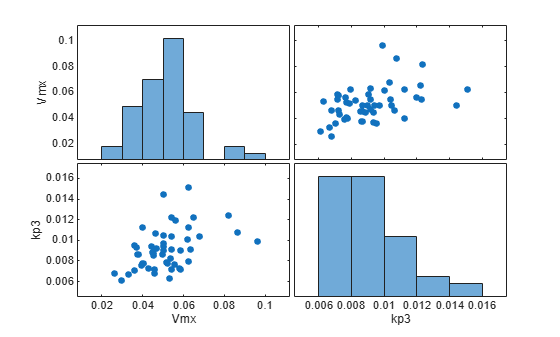Simulate the scenarios using the same SimFunction you created previously. You do not need to create a new SimFunction object even though the Scenarios object has been updated.

```sd2 = f(sObj,24); sbioplot(sd2);```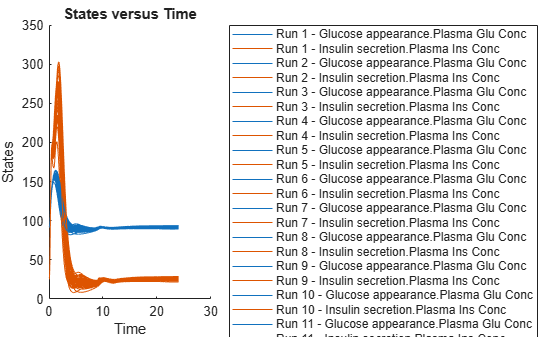By default, SimBiology uses the random sampling method. You can change it to the Latin hypercube sampling (or sobol or halton) for a more systematic space-filling approach.

`entry2struct = getEntry(sObj,2)`
```entry2struct = struct with fields: Name: {'Vmx' 'kp3'} Content: [2x1 prob.LognormalDistribution] Number: 50 RankCorrelation: [2x2 double] Covariance: [] SamplingMethod: 'random' SamplingOptions: [0x0 struct] ```
`entry2struct.SamplingMethod = 'lhs'`
```entry2struct = struct with fields: Name: {'Vmx' 'kp3'} Content: [2x1 prob.LognormalDistribution] Number: 50 RankCorrelation: [2x2 double] Covariance: [] SamplingMethod: 'lhs' SamplingOptions: [0x0 struct] ```

You can now use the updated structure to modify entry 2.

`updateEntry(sObj,2,entry2struct)`
```ans = Scenarios (50 scenarios) Name Content Number ____ ______________________ ______ Entry 1 dose SimBiology dose 1 x (Entry 2.1 Vmx Lognormal distribution 50 + Entry 2.2) kp3 Lognormal distribution 50 See also Expression property. ```

Visualize the sample values.

```sTbl2 = generate(sObj); [s,ax,bigax,h,hax] = plotmatrix([sTbl2.Vmx,sTbl2.kp3]); ax(1,1).YLabel.String = "Vmx"; ax(2,1).YLabel.String = "kp3"; ax(2,1).XLabel.String = "Vmx"; ax(2,2).XLabel.String = "kp3";```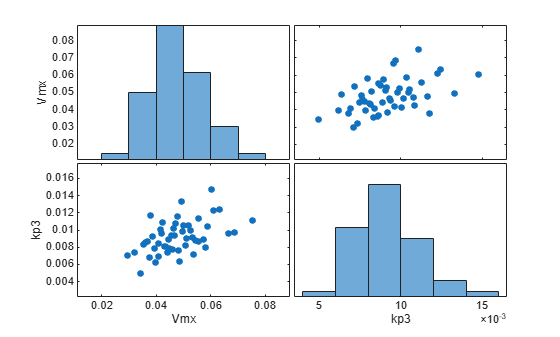Simulate the scenarios.

```sd3 = f(sObj,24); sbioplot(sd3);```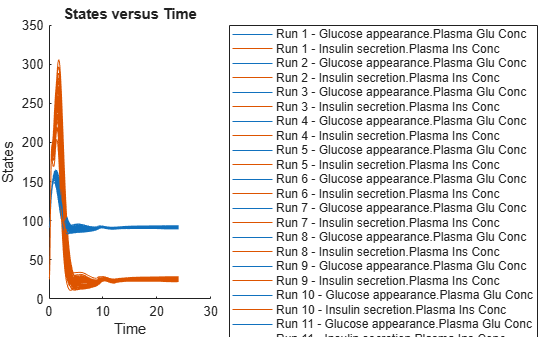Restore warning settings.

`warning(warnSettings);`

## Input Arguments

collapse all

Simulation scenarios, specified as a `SimBiology.Scenarios` object.

Entry name or index, specified as a character vector, string, or scalar positive integer. You can also specify the name of a subentry.

If you are specifying an index, it must be smaller than or equal to the number of entries in the object.

Data Types: `double` | `char` | `string`

Entry index, specified as a scalar positive integer. The entry index must be smaller than or equal to the number of entries in the object.

Data Types: `double`

Entry subindex, specified as a scalar positive integer. The subindex must be smaller than or equal to the number of subentries in the entry.

Data Types: `double`

## Output Arguments

collapse all

Simulation scenarios, returned as a `Scenarios` object.

The output `entryStruct` has the following fields:

• `Name` – Character vector or cell array of character vectors specifying the names of entries.

• `Content` – Vector of numeric values, dose objects, variant objects, or probability distribution objects.

If you query a random sampling entry without specifying a subentry, or if the random sampling entry references only one model component, `entryStruct` has the following additional fields:

• `Number` – Number of samples drawn from the distribution. If this field is empty `[]`, the number of samples is inferred from other entries. The default value is 2.

• `RankCorrelation` – Rank correlation matrix. This field is empty `[]` if no correlation matrix is specified.

• `Covariance` – Covariance matrix. This field is empty `[]` if no covariance matrix is specified.

• `SamplingMethod` – Character vector specifying the sampling method. Values are:

• `'random'` – Random sampling (default).

• `'lhs'` – Latin hypercube sampling.

• `'copula'` – Multivariate sample using a copula.

• `'sobol'` – Sobol quasirandom sample set.

• `'halton'` – Halton quasirandom sample set.

For details, see Sampling Methods.

### Topics

Introduced in R2019b

## SupportGet trial now